Next: Monte Carlo uncertainties Up: Time-of-flight analysis Previous: Time-of-flight analysis

### Fit results

We show in Fig. 8.21 a fit to the 3 T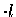data from Series A (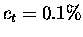) with the nominal physics input, where we obtained and confidence level 54.6%, with the normalization factor . The fit is very good, perhaps somewhat accidentally. But the situation is less perfect in the case for the 3 Tin series B (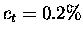) shown in Fig. 8.22, in which we obtained and CL 1.67% with .

The result of the formation rate scaling is presented in Fig. 8.23, where the total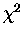is plotted against the log of the formation scaling parameter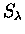. The log scaling of the horizontal axis was chosen to give relatively symmetrical shape of the resulting curve, compared to linear scaling. More physically motivated scalings (such as ) were tried, but none of them symmetrized the curves for both Series A and Series B simultaneously. The points near the minimum (indicated by filled squares) were fitted with a quadratic function to obtain the best fit value and its error was estimated from finding thein whichis increased by 1 from the minimum. The dotted line in the figure indicates reduced(i.e., ) of one. The results for two separate measurements (Series A and B) are given in Table 8.16. They are in an apparent disagreement by two standard deviations, indicating the existence of an unanticipated systematic uncertainty, which we shall discuss below.

The results for the resonant energy scaling are shown in Fig. 8.24. The horizontal axis is a linear scale here due to a reasonably symmetric distribution of the data points. A quadratic fit similar to above was performed to obtain the best fit, SE, and its error. Table 8.17 summarizes the energy scaling measurement. The results for two series of runs are in agreement within one standard deviation for the resonant energy measurements. Note that Series A had a higher sensitivity for both the rate and the energy measurements due to better statistics.

We note that, for both formation rate scaling and resonance energy scaling measurements, there are some uncertainties in the determination of the best fit value and its error due to the non quadratic shapes of thecurves. But these effects are relatively small compared to other uncertainties involved in the measurements.Next: Monte Carlo uncertainties Up: Time-of-flight analysis Previous: Time-of-flight analysis